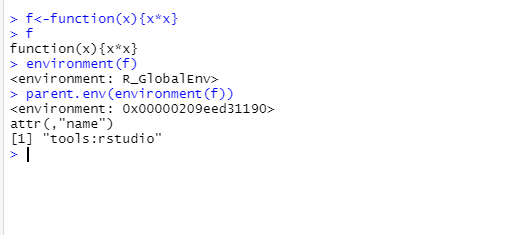Dynamic Scoping in R Programming

• Last Updated : 20 Jul, 2021

R is an open-source programming language that is widely used as a statistical software and data analysis tool. R generally comes with the Command-line interface. R is available across widely used platforms like Windows, Linux, and macOS. Also, the R programming language is the latest cutting-edge tool. The scoping rules for R are the main feature that makes it different from the original S language. R language uses lexical scoping or static scoping. A common alternative is Dynamic scoping.

Concept Behind Dynamic Scoping

Consider the following function:

R

 f <- function(x, y){   x^2 + y/z}

This function has 2 formal arguments x and y. In the body of the function, there is another symbol z. In this case, z is a free variable. The scoping rules of a language determine how values are assigned to free variables. Free variables are not formal arguments and are not local variables (assigned inside the body). Lexical scoping in R means that the values of free variables are searched for in the environment in which the function was defined. Searching for the value of the free variable means:

• If the value of the symbol is not found in the environment in which a function was defined, then the search is continued in the parent environment.
• The search continues down the sequence of the parent environment until the users hit the top-level environment; this usually the global environment (workspace) or the namespace of a package.
• If the value for a given symbol cannot be found once the empty environment has arrived, then an error is thrown. The value for the free variable is given in the below image.Typically a function is defined in the global environment so that the value of free variables is just found in the user’s workspace however in R one can have functions defined inside other functions. For example, have a look at the below code.

R

 make.power <- function(n){  pow <- function(x){    x = x^n  }  pow} cube <- make.power(3)square <- make.power(2)print(cube(3))print(square(3))

Output

 27
 9

The power function takes the argument x and raised the power n. So that makes the function pow return inside the function value. n is a free variable and is defines in the pow function. What’s in a function environment?

R

 ls(environment(cube))
 "n"   "pow"

R

 get("n", environment(cube))
 3

R

 ls(environment(square))
 "n"   "pow"

R

 get("n", environment(square))
 2

Let’s consider another example:

R

 y <- 10 # creating a function f which takes argument xf <- function(x){  # y is free variable  y <- 2      # g function is also a free variable  # not defined in the scope of function  y^2 + g(x) } g <- function(x){  # y is free variable  # value of y in this function is 10  x*y}

What is the value of y in this case?

• With the lexical scoping the value of y in the function g is looked up in the environment in which the function was defined, in this case, the global environment, so the value of y is 10.
• With the dynamic scoping the value of y is looked up in the environment from which the function was called (sometimes referred to as calling environment).
• In R the calling environment is known as the parent frame. So the value of y would be 2.

When a function is defined in the global environment and is subsequently called from the global environment, then the defining environment and the calling environment are the same. This can sometimes give the appearance of dynamic scoping.

R

 g <- function(x){  a <- 3  # in this case x is function a formal argument and  # a is local variable and  # y is free variable  x + a + y} # printing value of g(2)print(g(2))

If we call g(2) it will give an error like the following:

Output

After assigning value to y call g(2) as follows:

R

 g <- function(x){  a <- 3  x + a + y} # assigning value to yy <- 3 # print g(2)print(g(2))

Output

 8

My Personal Notes arrow_drop_up